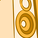# App Development

Announcements
Qlik Insider: SaaS capabilities for Data Integration and Analytics, May 25th! Register Now
cancel
Showing results for
Did you mean:Contributor

## Using Measure Variables in calculated expressions

Hi, I created some measure variables and want to complete some calculations.

The two in this example are mocccupancyexp_june & mdirectsalesjune.

At first I tried just dividing them (column 1), but realized it was incorrect. Columns 2 & 3 are the correct value & column 4 is a division of the two for the right percentage.

I was using =\$(moccupancyexp_june) / \$(mdirectsalesjune) for the initial calculation, but I must have made a mistake.  The image below has my calculations in the column header. Thanks for any helpLabels (1)
• ### expression

1 Solution

Accepted SolutionsMVP

Well, suppose mocccupancyexp_june is sum(DirectSales)+1 and mdirectsalesjune is sum(Amount). Then \$(moccupancyexp_june) / \$(mdirectsalesjune) would become sum(DirectSales)+1/sum(Amount). And that's completely different from (sum(DirectSales)+1) / sum(Amount). Just an example of what could go wrong. Since I don't know what expressions your variables really contain there's no telling what really is going wrong.

talk is cheap, supply exceeds demand
2 RepliesMVP

Well, suppose mocccupancyexp_june is sum(DirectSales)+1 and mdirectsalesjune is sum(Amount). Then \$(moccupancyexp_june) / \$(mdirectsalesjune) would become sum(DirectSales)+1/sum(Amount). And that's completely different from (sum(DirectSales)+1) / sum(Amount). Just an example of what could go wrong. Since I don't know what expressions your variables really contain there's no telling what really is going wrong.

talk is cheap, supply exceeds demandContributor
Author

I was missing a set of (). Thanks!